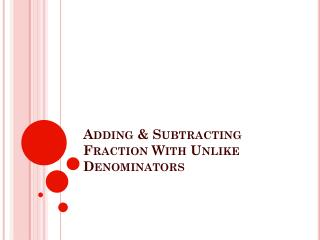DownloadDownload PresentationAdding & Subtracting Fraction With Unlike Denominators

# Adding & Subtracting Fraction With Unlike Denominators

Télécharger la présentation## Adding & Subtracting Fraction With Unlike Denominators

- - - - - - - - - - - - - - - - - - - - - - - - - - - E N D - - - - - - - - - - - - - - - - - - - - - - - - - - -
##### Presentation Transcript

1. Adding & Subtracting Fraction With Unlike Denominators

2. ex. ¼ + 2/3 • Step 1 • Rewrite the fractions using (LCD) lowest common denominator • Write the multiples of each denominator unitl a common one appears • Multiples of 3: 3, 6, 9, 12, 15... • Multiples of 4: 4, 8, 12, 16, 20... • The lowest common denominator of 3 and 4 is 12 • Multiply the numerator and the denominator of each fraction by the same factor • (1 x 3) = 3/12 (2 x 4) = 8/12 (4 x 3) (3 x 4)

3. Adding (ex. ¼ + 2/3) • Step 2 • Add the numerators of the fractions while keeping the denominators the same • 3/12 + 8/12 = (3 +8)/ 12 • 11/12 • Step 3 • Reduce the resulting fraction to lowest terms

4. How would we subtract? • Ex. 2/3 – ¼ • Multiples of 3 • Multiples of 4 • (2 x 4) = 8/12 (1 x 3) = 3/12 (3 x 4) (4 x 3) • 8/12 – 3/12= 5/12

5. The Butterfly Method